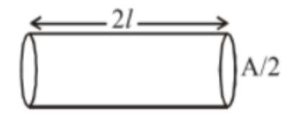# A conducting wire of lengthQuestion:

A conducting wire of length $^{\prime} l$, area of crosssection $\mathrm{A}$ and electric resistivity $\rho$ is connected between the terminals of a battery. A potential difference $\mathrm{V}$ is developed between its ends, causing an electric current. If the length of the wire of the same material is doubled and the area of cross-section is halved, the resultant current would be :

1. (1) $\frac{1}{4} \frac{\mathrm{VA}}{\rho l}$

2. (2) $\frac{3}{4} \frac{\mathrm{VA}}{\rho l}$

3. (3) $\frac{1}{4} \frac{\rho l}{\mathrm{VA}}$

4. (4) $4 \frac{\mathrm{VA}}{\rho l}$

Correct Option: 1

Solution:

(1)

As per the questionResistance $=\frac{\rho(2 l)}{(\mathrm{A} / 2)}=\frac{4 \rho l}{\mathrm{~A}}$

$\Rightarrow$ Current $=\frac{\mathrm{V}}{\mathrm{R}}=\frac{\mathrm{VA}}{4 \rho l}$